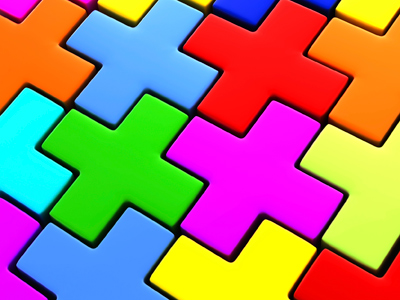Addition, plus, more than, total, altogether and sum are all words which mean add.

# Year 2 Calculation - Addition Language

This quiz addresses the requirements of the National Curriculum KS1 Maths and Numeracy for children aged 6 and 7 in year 2. Specifically this quiz is aimed at the section dealing with understanding the language of addition.

In Year 2, children extend their understanding of the language of addition to include the word sum. They should already know words such as add, addition, plus, more than, total and altogether. Recognising and understanding the language of addition is essential in order to be able to make decisions about which operation to use to solve calculations and problems.

Question 1
Which word could be used in this number sentence?
7 ___ 5 = 12
plus
minus
subtract
multiplied by
Question 2
What is the sum of 7, 9 and 3?
17
23
18
19
To find 'the sum' add all the numbers together
Question 3
What is the total of 12, 3 and 5?
25
18
20
19
To find the total, add all the numbers together
Question 4
15 is the sum of which 2 numbers?
6 and 9
11 and 3
15 and 4
9 and 8
The sum of the numbers is what you find when they are added together
Question 5
What is the symbol for addition?
-
+
=
÷
Question 6
38 is the total of which 2 numbers?
80 and 3
20 and 4
30 and 8
3 and 8
To find the total, add all the numbers together
Question 7
How much is 6 more than 8?
28
68
12
14
'More than' means counting on from one number
Question 8
Which word could be used in this number sentence?
9 ___ 6 = 15
take away
subtract
divided by
To add means to count up the total of all the numbers together
Question 9
Which of the following is not an addition word?
Sum
Division
Altogether
Total
Division is not adding or finding a total or sum
Question 10
I have 4 cakes, 5 buns and 2 biscuits. How many treats do I have altogether?
7
9
11
15
The clue word in the problem is 'altogether'